- F_un mathematics - http://cage.ugent.be/~kthas/Fun -

The skeleton of SoulÃ©’s F_un geometry

Posted By javier On October 4, 2008 @ 6:23 pm In featured,graduate,Soule2004 | No CommentsThe development of a succesful geometry over the nonexistent field with one elementrequires the creation of appropriate categories to work with. So far, we have seen as a part of thefolklore  that we have a nice category of vector spaces over our dearest phantom field, and how this category of vector spaces gives a way to develop an absolute linear algebra . In that linear algebra theory, a fundamental rÃ´le is played by the finite (abelian) field extensions.

Christophe Soule  tried in his 2004 paper Les varietes sur le corp a un element  to take advantage of these field extensions in order to develop a first approach to an algebraic geometry over. His main guiding idea is stated right at the begining of the paper:

On part de l’idÃ©e qu’une varietÃ©(de type fini) surdoit avoir une extension des scalaires Ã, qui sera un schÃ©made type fini surWhich means

We start from the idea that a variety(of finite type) overmust admit an extension of scalars to, which will be an schemeof finite type overSince one of our mantras is that we should avoid hitting directly on the elusive, trying to work over collections of objects defined over it, the most appropriate reformulation of the notion of scheme  that we may use is the, shortest ever, definition (of course equivalent to the classical one):

An scheme over a ground field (or ring)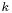is a covariant functor from the category of-algebras to the category of sets which is locally representable  by an algebra.

With this definition, if we have an schemeover, given by the functor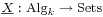, and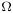a-algebra, the extension of scalars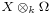satisfy that for every-algebrawe have an inclusion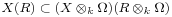satisfying certain universal property. If we want to do the same thing within the place ofandin the place of, we need to find out at least a big enough collection of rings defined over the field with one element in order to have something to apply our functor! Now, even if it is not very clear what should we take as the category of algebras over(I have my own feelings about this, but will ellaborate on that some other day) there is one thing that we can certainly agree: the field extensionsshould be the first candidates to belong to such category.

Now is when wishful thinking comes into play. Since these field extensions are thought of as “adding roots of 1″, the extension of scalarsshould have rank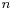over the integers, and that hints that the following equation should be true: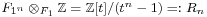In particular, for any hypothetical varietyoverwe must have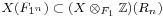Going a bit further, we shall assume that the extension of scalars fromtoinduces an equivalence of categories between some (yet to be defined) category of algebras overand the category of rings flat and of finite type over, i.e. rings, whose underlying additive group is a lattice of finite rank.

With this assumption in mind, we have all the ingredients we need in order to start giving a definition of what a variety overshould be. But that will come in the next post!

URL to article: http://cage.ugent.be/~kthas/Fun/index.php/the-skeleton-of-soules-f_un-geometry.html

URLs in this post:

 Image: http://cage.ugent.be/~kthas/Fun/index.php/the-f_un-folklore.html

 absolute linear algebra: http://cage.ugent.be/~kthas/Fun/index.php/f_un-linear-algebra.html

 Christophe Soule: http://www.ihes.fr/~soule/

 Les varietes sur le corp a un element: http://cage.ugent.be/~kthas/Fun/index.php/soule2004.html

 scheme: http://en.wikipedia.org/wiki/Scheme_(mathematics)

 representable: http://en.wikipedia.org/wiki/Representable_functor